## How to Calculate and Solve for Relaxation Modulus | Polymer

The relaxation modulus is represented by the image below.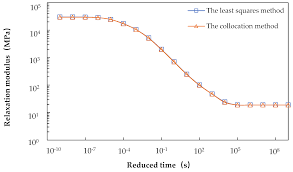To compute for relaxation modulus, two essential parameters are needed and these parameters are Time Dependent Stress (σ(t)) and Strain Level (εo).

The formula for calculating relaxation modulus:

Er(t) = σ(t)/εo

Where:

Er(t) = Relaxation Modulus
σ(t) = Time Dependent Stress
εo = Strain Level

Let’s solve an example;
Find the relaxation modulus when the time dependent stress is 12 and the strain level is 6.

This implies that;

σ(t) = Time Dependent Stress = 12
εo = Strain Level = 6

Er(t) = 12/6
Er(t) = 2

Therefore, the relaxation modulus is 2 Pa.

Calculating the Time Dependent Stress when the Relaxation Stress and the Strain Level is Given.

σ(t) = Er(t)o)

Where:

σ(t) = Time Dependent Stress
Er(t) = Relaxation Modulus
εo = Strain Level

Let’s solve an example;
Find the time dependent stress when the relaxation modulus is 18 and the strain level is 5.

This implies that;

Er(t) = Relaxation Modulus = 18
εo = Strain Level = 5

σ(t) = Er(t)o)
σ(t) = 18 (5)
σ(t) = 90

Therefore, the time dependent stress is 90.

## How to Calculate and Solve for Tensile Strength of Polymers | Polymer

The tensile strength of polymers is represented by the image below.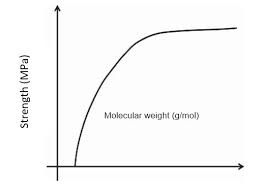To compute for tensile strength of polymers, three essential parameters are needed and these parameters are Tensile Strength at Infinite Weight (TS), Constant (A) and Mean Number Molecular Weight (M’n).

The formula for tensile strength of polymers:

TS = TS – (A/M’n)

Where:

TS = Tensile Strength of Polymers
TS = Tensile Strength at Infinite Weight
A = Constant
M’n = Mean Number Molecular Weight

Let’s solve an example;
Find the tensile strength of polymers when the tensile strength at infinite weight is 8, the constant is 4 and the mean number molecular weight is 2.

This implies that;

TS = Tensile Strength at Infinite Weight = 8
A = Constant = 4
M’n = Mean Number Molecular Weight = 2

TS = (8) – (4/2)
TS = (8) – (2)
TS = 6

Therefore, the tensile strength of polymers is 6 Pa.

Calculating the Tensile Strength at Infinite Weight when the Tensile Strength of Polymers, Constant and the Mean Number Molecular Weight is Given.

TS = TS – (A / M’n)

Where:

TS = Tensile Strength at Infinite Weight
TS = Tensile Strength of Polymers
A = Constant
M’n = Mean Number Molecular Weight

Given an example;
Find the tensile strength at infinite weight when the tensile strength of polymers is 44, the constant is 80 and the mean number molecular weight is 10.

This implies that;

TS = Tensile Strength of Polymers = 44
A = Constant = 80
M’n = Mean Number Molecular Weight = 10

TS = TS – (A / M’n)
TS = 44 – (80 / 10)
TS = 44 – 8
TS = 36

Therefore, the tensile strength at infinite weight is 36.

## How to Calculate and Solve for Solubility of Polymer | Polymer

The solubility of polymer is represented by the image below.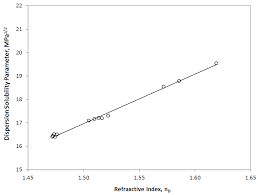To compute for solubility of polymer, two essential parameters are needed and these parameters are Concentration of Diffusing Specie in Polymer (C) and Partial Pressure (P).

The formula for calculating solubility of polymer:

S = C/P

Where:

S = Solubility of Polymer
C = Concentration of Diffusing Specie in Polymer
P = Partial Pressure

Let’s solve an example;
Find the solubility of polymer when the concentration of diffusing specie in polymer is 21 and the partial pressure is 3.

This implies that;

C = Concentration of Diffusing Specie in Polymer = 21
P = Partial Pressure = 3

S = 21/3
S = 7

Therefore, the solubility of polymer is 7 Pa.

Calculating the Concentration of Diffusing Specie when the Solubility of Polymer and the Partial Pressure is Given.

C = PS

Where:

C = Concentration of Diffusing Specie in Polymer
S = Solubility of Polymer
P = Partial Pressure

Let’s solve an example;
Find the concentration of diffusing specie in polymer when the solubility of polymer is 10 and the partial pressure is 4.

This implies that;

S = Solubility of Polymer = 10
P = Partial Pressure = 4

C = PS
C = 10 x 4
C = 40

Therefore, the concentration of diffusing specie is 40.

## How to Calculate and Solve for Permeability Coefficient | Polymer

The permeability coefficient is represented by the image below.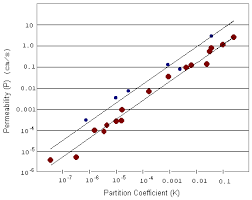To compute for permeability coefficient, two essential parameters are needed and these parameters are Diffusion Coefficient (D) and Solubility of the Polymer (S).

The formula for calculating the permeability coefficient:

Pm = DS

Where;

Pm = Permeability Coefficient
D = Diffusion Coefficient
S = Solubility of the Polymer

Let’s solve an example;
Given that the diffusion coefficient is 24 and the solubility of the polymer is 12. Find the permeability coefficient?

This implies that;

D = Diffusion Coefficient = 24
S = Solubility of the Polymer = 12

Pm = (24)(12)
Pm = 288

Therefore, the permeability coefficient is 288 Kg/msPa.

Calculating the Diffusion Coefficient when the Permeability Coefficient and the Solubility of the Polymer is Given.

D = Pm / S

Where;

D = Diffusion Coefficient
Pm = Permeability Coefficient
S = Solubility of the Polymer

Given an example;
Find the diffusion coefficient when the permeability coefficient is 30 and the solubility of the polymer is 5.

This implies that;

Pm = Permeability Coefficient = 30
S = Solubility of the Polymer = 5

D = Pm / S
D = 30 / 5
D = 6

Therefore, the diffusion coefficient is 6.

## How to Calculate and Solve for Percentage Crystallinity | Polymer

The percentage crystallinity is represented by the image below.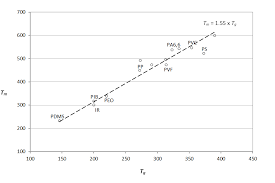To compute for the percentage crystallinity, three essential parameters are needed and these parameters are Density of Amorphous Material (ρa), Density of Specimen (ρs) and Density of Perfect Crystalline Polymer (ρc).

The formula for calculating percentage crystallinity:

%C = 100(ρcs – ρa)/ρs(ρc – ρa))

Where:

%C = Percentage Crystallinity
ρa = Density of Amorphous Material
ρs = Density of Specimen
ρc = Density of Perfect Crystalline Polymer

Let’s solve an example;
Find the percentage crystallinity where the density of amorphous material is 12, the density of specimen is 10 and the density of perfect crystalline polymer is 4.

This implies that;

ρa = Density of Amorphous Material = 12
ρs = Density of Specimen = 10
ρc = Density of Perfect Crystalline Polymer = 4

%C = 100(4(10 – 12)/10(4 – 12))
%C = 100(4(-2)/12(-8))
%C = 100((-8)/(-80))
%C = 100(0.1)
%C = 10

Therefore, the percentage crystallinity is 10%.

## How to Calculate and Solve for Average Repeat Unit Molecular Weight for Co-Polymers | Polymer

The average repeat unit molecular weight for co-polymers is represented by the image below.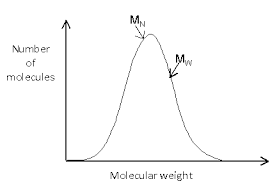To compute for average repeat unit molecular weight for co-polymers, one essential parameter is needed and the parameter is Numbers of co-polymers (n). By determining the number of co-polymers, four parameters is needed to get your answer so the parameters are Mole Fraction (f1), Molecular Weight (M1), Mole Fraction (f2) and Molecular Weight (M2).

The formula for calculating the average repeat unit molecular weight for co-polymers:

m’ = ΣfiMi

Let’s solve an example;
Find the average repeat unit molecular weight for co-polymers when the mole fraction(s) is 8,2 and the molecular weight(s) is 4,6.

This implies that;

m’ = ΣfiMi

Where:

m’ = Average Repeat Unit Molecular Weight for Co-Polymers
fi = Mole Fraction(s)
Mi = Molecular Weight(s)

m’ = (8)(4) + (2)(6)
m’ = (32) + (12)
m’ = 44

Therefore, the average repeat unit molecular weight for co-polymers is 44.

## How to Calculate and Solve for Degree of Polymerization | Polymer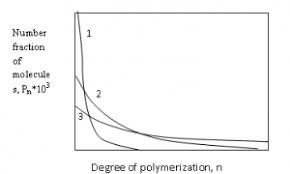The image of degree of polymerization is represented above.

To compute for degree of polymerization, two essential parameters are needed and these parameters are Mean Number Molecular Weight (M’nand Unit Molecular Weight (m).

The formula for calculating degree of polymerization:

D.P. = M’n/m

Where:

D.P. = Degree of Polymerization
M’n = Mean Number Molecular Weight
m = Unit Molecular Weight

Let’s solve an example;
Find the degree of polymerization when the mean number molecular weight is 28 and the unit molecular weight is 4.

This implies that;

M’n = Mean Number Molecular Weight = 28
m = Unit Molecular Weight = 4

D.P. = 28/4
D.P. = 7

Therefore, the degree of polymerization is 7.

Calculating the Mean Number Molecular Weight when the Degree of Polymerization and the Unit Molecular Weight is Given.

M’n = D.P (m)

Where;

M’n = Mean Number Molecular Weight
D.P. = Degree of Polymerization
m = Unit Molecular Weight

Let’s solve an example;
Find the mean number molecular weight when the degree of polymerization is 6 and the unit molecular weight is 4.

This implies that;

D.P. = Degree of Polymerization = 6
m = Unit Molecular Weight = 4

M’n = D.P (m)
M’n = 6 (4)
M’n = 24

Therefore, the degree of polymerization is 24.

## How to Calculate and Solve for Number Average Molecular Weight | Polymer

The image of mean is represented below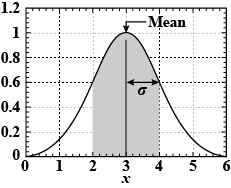To compute for mean, two essential parameters are needed and these parameters are Set of data and Corresponding frequencies accordingly.

The formula for calculating mean:

Mean = ∑fx / ∑f

Where;

∑fx = Summation of the product of the data and its corresponding frequency.
∑f = Summation of the frequencies

Let’s solve an example;
Find the mean when the set of data is 12 and the corresponding frequencies accordingly is 2.

This implies that;

x f (fx) fx
12 2 (12 x 2) 24

∑fx = 24
∑f = 2

Mean = ∑fx / ∑f
Mean = 24 / 2
Mean = 12

Therefore, the mean is 12.